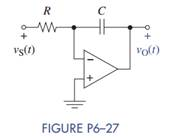### Create an Account

Home / Questions / The OP AMP integrator in Figure P6–27 has R = 22 kΩ C 0 001 μF and vO 0 0 V The input is...

# The OP AMP integrator in Figure P6–27 has R = 22 kΩ C 0 001 μF and vO 0 0 V The input is vS t 2 sin ωt u t V Derive an expression for vO t and find the smallest allowable value of

The OP AMP integrator in Figure P6–27 has R = 22 kΩ, C = 0.001 μF, and vO(0) = 0 V. The input is vS(t) = 2 sin (ωt) u (t) V. Derive an expression for vO(t) and find the smallest allowable value of ω for linear operation of the OP AMP. Assume VCC = ±15 V.Jun 15 2020 View more View LessSubscribe To Get Solution